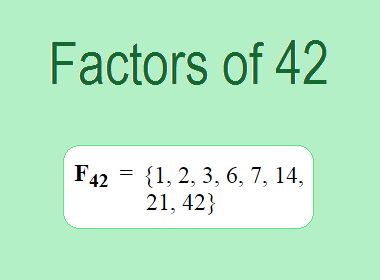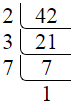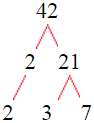# Factors of 42The factors of 42 are 1, 2, 3, 6, 7, 14, 21, and 42 i.e. F42 = {1, 2, 3, 6, 7, 14, 21, 42}. The factors of 42 are all the numbers that can divide 42 without leaving a remainder.

We can check if these numbers are factors of 42 by dividing 42 by each of them. If the result is a whole number, then the number is a factor of 42. Let's do this for each of the numbers listed above:

·        1 is a factor of 42 because 42 divided by 1 is 42.

·        2 is a factor of 42 because 42 divided by 2 is 21.

·        3 is a factor of 42 because 42 divided by 3 is 14.

·        6 is a factor of 42 because 42 divided by 6 is 7.

·        7 is a factor of 42 because 42 divided by 7 is 6.

·        14 is a factor of 42 because 42 divided by 14 is 3.

·        21 is a factor of 42 because 42 divided by 21 is 2.

·        42 is a factor of 42 because 42 divided by 42 is 1.

## How to Find Factors of 42?

1 and the number itself are the factors of every number. So, 1 and 42 are two factors of 42. To find the other factors of 42, we can start by dividing 42 by the numbers between 1 and 42. If we divide 42 by 2, we get a remainder of 0. Therefore, 2 is a factor of 42. If we divide 42 by 3, we get a remainder of 0. Therefore, 3 is also a factor of 42.

Next, we can check if 4 is a factor of 42. If we divide 42 by 4, we get a remainder of 2. Therefore, 4 is not a factor of 42. We can continue this process for all the possible factors of 42.

Through this process, we can find that the factors of 42 are 1, 2, 3, 6, 7, 14, 21, and 42. These are the only numbers that can divide 42 without leaving a remainder.

********************

********************

## Properties of the Factors of 42

The factors of 42 have some interesting properties. One of the properties is that the sum of the factors of 42 is equal to 96. We can see this by adding all the factors of 42 together:

1 + 2 + 3 + 6 + 7 + 14 + 21 + 42 = 96

Another property of the factors of 42 is that the prime factors of 42 are 2, 3, and 7.

## Applications of the Factors of 42

The factors of 42 have several applications in mathematics. One of the applications is in finding the highest common factor (HCF) of two or more numbers. The HCF is the largest factor that two or more numbers have in common. For example, to find the HCF of 42 and 30, we need to find the factors of both numbers and identify the largest factor they have in common. The factors of 42 are 1, 2, 3, 6, 7, 14, 21, and 42. The factors of 30 are 1, 2, 3, 5, 6, 10, 15, and 42. The largest factor that they have in common is 6. Therefore, the HCF of 42 and 30 is 6.

Another application of the factors of 42 is in prime factorization. Prime factorization is the process of expressing a number as the product of its prime factors. The prime factors of 42 are 2, 3, and 7, since these are the only prime numbers that can divide 42 without leaving a remainder. Therefore, we can express 42 as:

42 = 2 × 3 × 7

We can do prime factorization by division and factor tree method also. Here is the prime factorization of 42 by division method,42 = 2 × 3 × 7

Here is the prime factorization of 42 by the factor tree method,42 = 2 × 3 × 7

## Conclusion

The factors of 42 are the numbers that can divide 42 without leaving a remainder. The factors of 42 are 1, 2, 3, 6, 7, 14, 21, and 42. The factors of 42 have some interesting properties, such as having a sum of 96. The factors of 42 have several applications in mathematics, such as finding the highest common factor and prime factorization.